×

Search anything:

# Iterative Deepening Search

#### Search Algorithms Algorithms Graph AlgorithmsGet this book -> Problems on Array: For Interviews and Competitive Programming

In this article, we are going to discuss about the Iterative Deepening Search Technique. It is also, known as Iterative Deepening Depth-First Search ( IDDFS) and is a modification of Depth First Search and Depth Limited Search.

1. Recap of DFS and BFS
2. Drawbacks of DFS and BFS
3. Depth-Limited Search (DLS)
4. Implementation of DLS
5. Iterative Deepening Depth-First Search ( IDDFS)
6. Implementation of IDDFS
7. Time Complexity
8. Comparison between DFS, DLS and IDDFS
9. Conclusion

The main goal of any graph search traversal technique is to find the shortest possible path between a source node and a goal node.

You are probably familiar with the Depth-First Search and the Breadth-First Search technique for graph traversal.

Let's revisit the concepts quickly:

## 1. DFS and BFS

Depth-First Search is a graph traversal technique that explores the graph depth-wise. It starts with a source node, explores one of its neighboring node and then explores the neighbor of this node. Once all the neighbor nodes of the current node are explored, the algorithm backtracks and continues this process until it has reached the desired node or explored all the nodes in the given graph at least once.

To know more about DFS, refer to DFS implementation

Breadth-First Search is a graph traversal technique that explores the graph layer-wise. It starts with a source node and explores all its adjacent nodes before moving to the next layer.

The following picture illustrates the DFS and BFS graph traversal: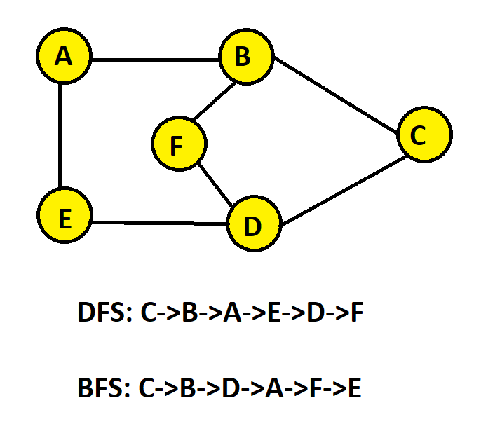## 2. Drawbacks of DFS and BFS

Both DFS and BFS have certain limitations as highlighted in the table below: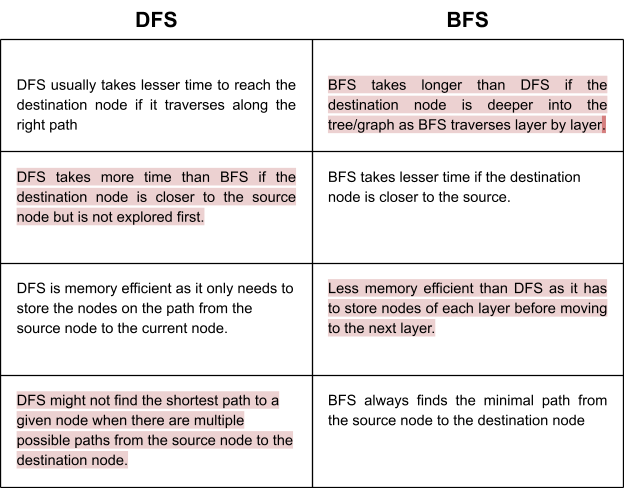Consider the case of a search tree with an unknown (infinite) depth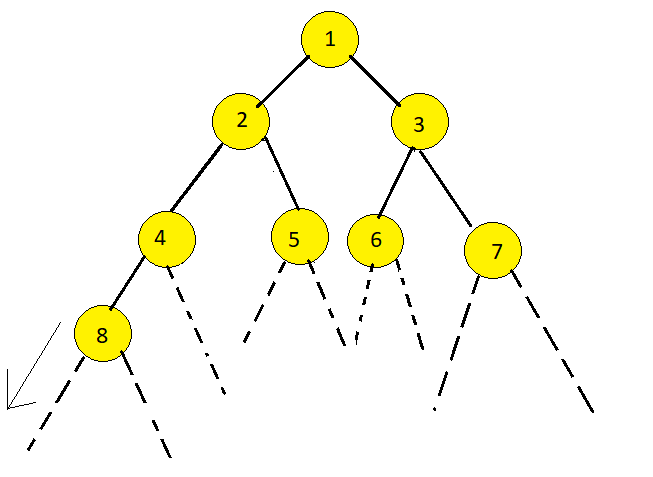Using DFS technique, we might not ever reach the goal node. DFS may get stuck in an infinite loop.

To overcome the shortcomings of DFS, we may use the depth-limited search technique.

## 3. Depth Limited Search

A depth-limited search (DLS) algorithm is similar to DFS with a depth limit i.e it only explores the nodes up to a finite depth, say d. The algorithm treats the nodes at depth d, as if they don't have any successor nodes.

Consider a case where depth-limit d=2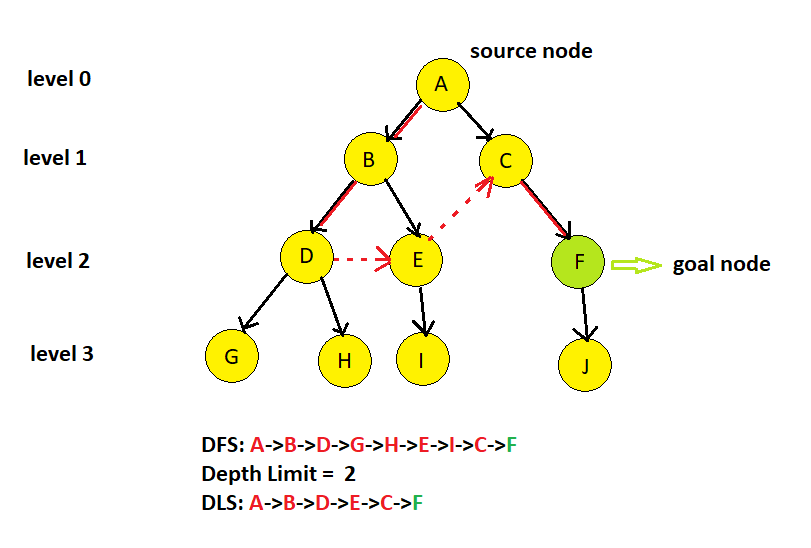The Depth Limited Search arrives at the goal node faster if the goal node is at a depth equal to d, i.e in shortest possible path.

If the goal node is at a depth lesser than d, the suboptimal path may be arrived at whereas in the case of depth being greater than d, the goal node will be unreachable.

• Depth-limited search overcomes the drawback of the infinite path in DFS. [Note that DFS is a special case where the depth limit is infinity]

• DLS fails in the following two cases:

1. The goal node is beyond the depth limit.
2. There is no path as the goal node does not exist in the graph/tree.

## 4. Implementation of Depth-Limited Search

Given below is the recursive implementation of DLS.
The search terminates if limit<0 or if the goal node does not exist within the depth limit.

``````#include <bits/stdc++.h>
using namespace std;

class Graph {
public:

void addEdge(int v, int w)
{
}

int DLS(int v, int g,int l);

};

int Graph::DLS(int v,int goal,int limit)
{
if(v==goal)
{
return 1;
}
for (auto i = adj[v].begin(); i != adj[v].end(); ++i)
{
if(limit-1>=0)
{
if(DLS(*i,goal,limit-1)!=-1)
return 1;
}
}
return -1;

}

int main()
{
int goal,limit;
Graph g;

cout<<"Enter the goal node: ";
cin>>goal;
cout<<"Enter depth limit: ";
cin>>limit;

int res=g.DLS(0,goal,limit);

if(res==-1)
else
cout<<"Goal node found within depth limit";

return 0;
}
``````

## 5. Iterative Deepening Depth-First Search (IDDFS)

The Iterative Deepening Depth-First Search (or Iterative Deepening search) algorithm, repeatedly applies depth-limited search with increasing limits.

It gradually increases limits from 0,1,...d, until the goal node is found.

It terminates in the following two cases:

1. When the goal node is found
2. The goal node does not exist in the graph/tree.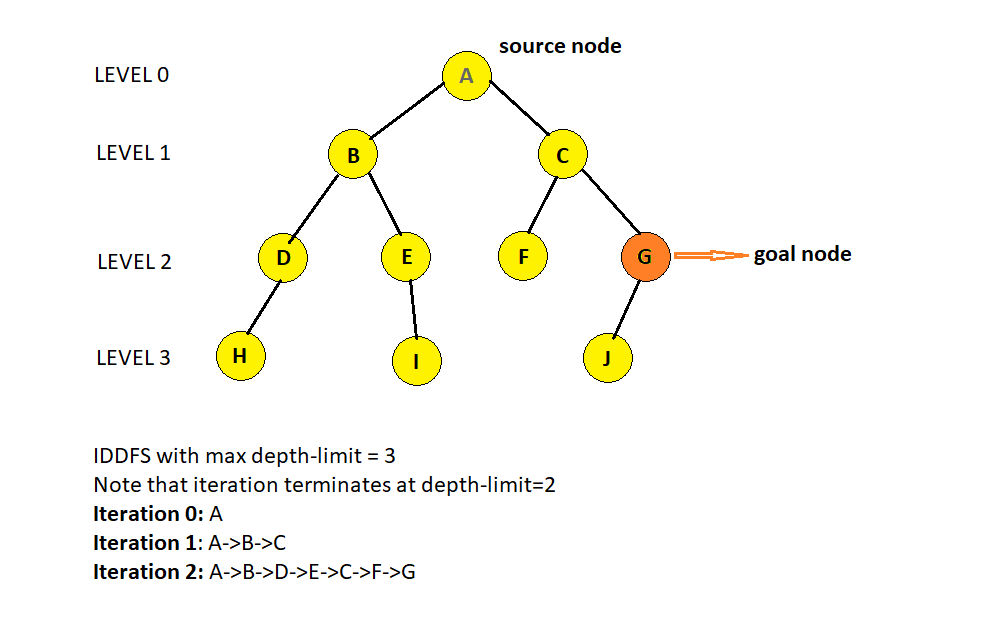## 5. Implementation of IDDFS

``````#include <bits/stdc++.h>
using namespace std;

class Graph {
public:

void addEdge(int v, int w)
{
}

int DLS(int v, int g,int l);

int IDDFS(int v,int g,int l);
};

int Graph::DLS(int v,int goal,int limit)
{
if(v==goal)
{
return 1;
}
for (auto i = adj[v].begin(); i != adj[v].end(); ++i)
{

if(limit-1>=0)
{
cout<<*i<<" ";
if(DLS(*i,goal,limit-1)!=-1)
return 1;
}
}
return -1;

}

int Graph::IDDFS(int src, int goal, int limit)
{
for(int i=0;i<=limit;i++)
{
cout<<"Iteration "<<i<<": "<<src<<" ";
if(DLS(src,goal,i)==1)
{
return 1;
}
cout<<"\n";
}
return -1;
}

int main()
{
Graph gp;

int src=0, goal=9, limit=3;

if(gp.IDDFS(src,goal,limit)==1)
cout<<"\nGoal node found within depth limit";
else

return 0;
}

``````

### Output

``````Iteration 0: 0
Iteration 1: 0 1 2
Iteration 2: 0 1 3 4 2 5 6
Iteration 3: 0 1 3 7 4 2 5 8 6 9
Goal node found within depth limit
``````

IDDFS overcomes the drawbacks of dfs and bfs discussed previously. It combines the space-efficiency of DFS - O(bd) and time-efficiency of BFS algorithm - O(b^d).

## 7. Time Complexity of IDDFS

Branching factor (b): The average number of children of each node.

If b is the branching factor, and d is the depth of the goal node or the depth at which the iteration of IDDFS function terminates, the time complexity is O(b^d) and space complexity is O(bd).

Explanation:
The total number of nodes generated in the worst case is
b(d) + b^2(d-1) + .......b^d(1).

## Comparison between DFS,DLS and IDDFS

The criteria for evaluating the performance of a search algorithm are completeness, optimality, time and space complexity.

Complete - A search algorithm is complete if it guarantees to find the goal node if it exists.

Optimal - A search algorithm is optimal if it finds the shortest path from the source node.

Time Complexity - The time taken to complete the search.

Space Complexity - The memory requirement of the search algorithm.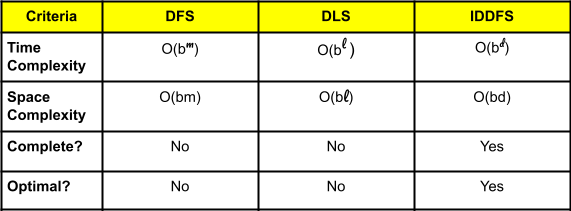Here l = depth-limit, d = depth of the goal node, m = depth of the search tree/graph.

## Conclusion

With this article at OpenGenus, you now have a complete understanding of the Iterative Deepening Depth-First Search (IDDFS) algorithm, and how it compares with the Depth-First Search (DFS), Breadth-First Search (BFS) and Depth-Limited Search (DLS).